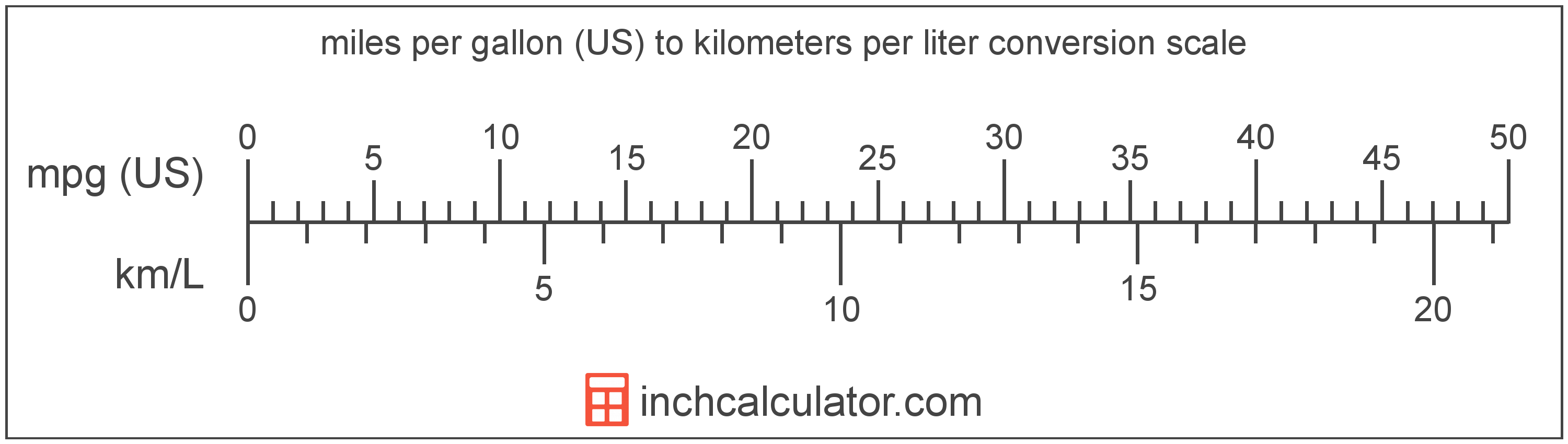# Miles per Gallon (US) to Kilometers per Liter Converter

Enter the fuel economy in miles per gallon (US) below to get the value converted to kilometers per liter.

Results in Kilometers per Liter:1 mpg (US) = 0.425144 km/L

Do you want to convert kilometers per liter to miles per gallon (US)?

## How to Convert Miles per Gallon (US) to Kilometers per Liter

To convert a measurement in miles per gallon (US) to a measurement in kilometers per liter, multiply the fuel economy by the following conversion ratio: 0.425144 kilometers per liter/mile per gallon (US).

Since one mile per gallon (US) is equal to 0.425144 kilometers per liter, you can use this simple formula to convert:

kilometers per liter = miles per gallon (US) × 0.425144

The fuel economy in kilometers per liter is equal to the fuel economy in miles per gallon (US) multiplied by 0.425144.

For example, here's how to convert 5 miles per gallon (US) to kilometers per liter using the formula above.
kilometers per liter = (5 mpg (US) × 0.425144) = 2.125719 km/LMiles per gallon (US) and kilometers per liter are both units used to measure fuel economy. Keep reading to learn more about each unit of measure.

## What Are Miles per Gallon (US)?

Miles per gallon is the distance traveled in miles consuming just one gallon of fuel.

When evaluating fuel efficiency, the more miles per gallon, the more fuel efficient a vehicle is and the less fuel consumed. Thus, the higher the mpg rating of a vehicle the less fuel it will consume.

Miles per gallon (US) can be abbreviated as mpg (US), and are also sometimes abbreviated as mpg. For example, 1 mile per gallon (US) can be written as 1 mpg (US) or 1 mpg.

## What Are Kilometers per Liter?

Kilometers per liter is the distance that can be traveled in kilometers using one liter of fuel.

Kilometers per liter can be abbreviated as km/L, and are also sometimes abbreviated as kpl or kmpl. For example, 1 kilometer per liter can be written as 1 km/L, 1 kpl, or 1 kmpl.

In the expressions of units, the slash, or solidus (/), is used to express a change in one or more units relative to a change in one or more other units.

## Mile per Gallon (US) to Kilometer per Liter Conversion Table

Table showing various mile per gallon (US) measurements converted to kilometers per liter.
Miles Per Gallon (US) Kilometers Per Liter
1 mpg (US) 0.425144 km/L
2 mpg (US) 0.850288 km/L
3 mpg (US) 1.2754 km/L
4 mpg (US) 1.7006 km/L
5 mpg (US) 2.1257 km/L
6 mpg (US) 2.5509 km/L
7 mpg (US) 2.976 km/L
8 mpg (US) 3.4012 km/L
9 mpg (US) 3.8263 km/L
10 mpg (US) 4.2514 km/L
11 mpg (US) 4.6766 km/L
12 mpg (US) 5.1017 km/L
13 mpg (US) 5.5269 km/L
14 mpg (US) 5.952 km/L
15 mpg (US) 6.3772 km/L
16 mpg (US) 6.8023 km/L
17 mpg (US) 7.2274 km/L
18 mpg (US) 7.6526 km/L
19 mpg (US) 8.0777 km/L
20 mpg (US) 8.5029 km/L
21 mpg (US) 8.928 km/L
22 mpg (US) 9.3532 km/L
23 mpg (US) 9.7783 km/L
24 mpg (US) 10.2 km/L
25 mpg (US) 10.63 km/L
26 mpg (US) 11.05 km/L
27 mpg (US) 11.48 km/L
28 mpg (US) 11.9 km/L
29 mpg (US) 12.33 km/L
30 mpg (US) 12.75 km/L
31 mpg (US) 13.18 km/L
32 mpg (US) 13.6 km/L
33 mpg (US) 14.03 km/L
34 mpg (US) 14.45 km/L
35 mpg (US) 14.88 km/L
36 mpg (US) 15.31 km/L
37 mpg (US) 15.73 km/L
38 mpg (US) 16.16 km/L
39 mpg (US) 16.58 km/L
40 mpg (US) 17.01 km/L